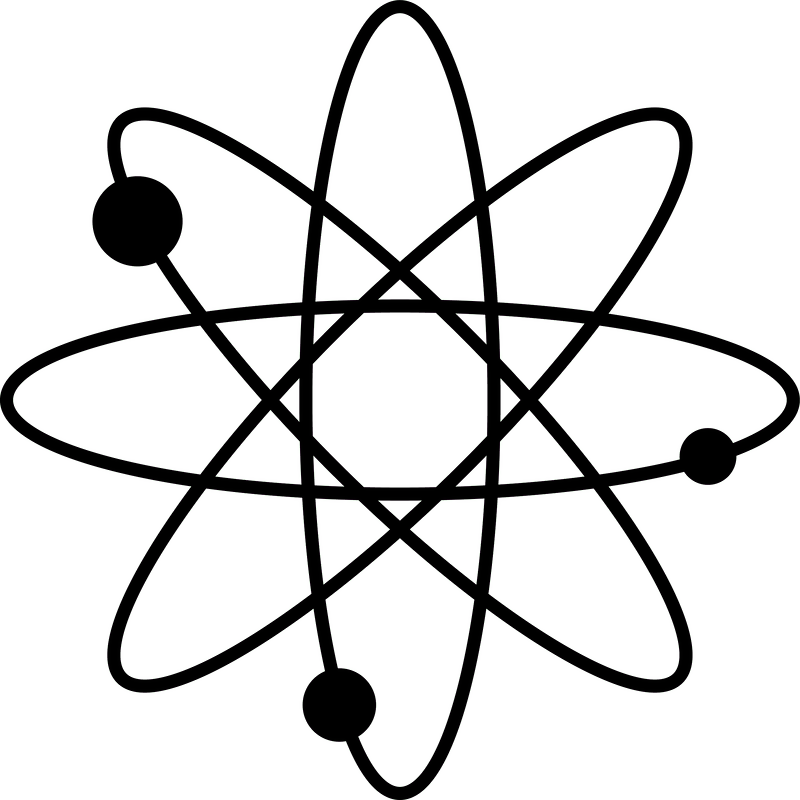What makes an Equation?

What makes an equation?

In school one often thinks about equations as formulas. The aim of a formula is to shorten or condense information into an expression so that you don't need to worry about the derivation. You want to know the volume of a sphere, you look for the right formula (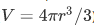), you plug in the value for the radius, you plug in the value for π, and you are done.

The purpose of a formula is that you don't need to worry about it. Once you know what formula to use things are easy, and you can worry about a different part of the problem.

Equations (as we will look at them in this course) are different. Equations are something you want to contemplate and to understand how they were derived. Their fundamental role is not to shorten your computations, but to express a relation, to equate two physical quantities.

方程式（我们在这门课中看他们）是不同的。方程是你想思考并理解它们是如何派生出来的东西。它们的基本作用不是缩短计算，而是表达一个关系，使两个物理量相等。

The easiest and most accessible way of contemplating an equation is to think about its dimensions. On both sides of the equal sign you need to have the same dimensions. Let's take one of the most famous equations in physics (we will talk about a way of deriving it in Lesson 3).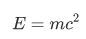We know that E is an energy, so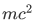must have dimensions of energy. If you recall from high school that the kinetic energy of a particle is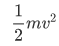then this is not surprising. Because if: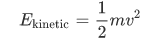Then: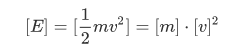But what if you can't remember that result? Then you would need to start from a quantity that you consider to be the definition of energy. Here, you can play Newton and construct your own mechanics. You can intuit that energy has to do with force, but it is not equal to force. You need to use energy to lift a heavy body. The heavier the body or the higher the lift, the more energy you need to use. So you could expect that the energy would be proportional to the weight of the body (i.e. the force) and the height over which you are lifting (i.e. the distance). So you could write something like:

energy=force×distance

Now, you might worry that not all the energy you put in is used for lifting the body. Maybe something is lost through friction, and you should add something. Or maybe only a fraction of the energy you put in is being used, so you need multiply by some number. So maybe you will write:

energy you put in=1.1×force×distance

Or

energy you put in=force×distance+frictionforce×some distance

But either way, the dimensions would be the same. Notice that if you think that some energy is lost through friction, you cannot simply add a factor corresponding to the friction forces. You need to multiply the force by some factor that has dimensions of distance, otherwise you would be adding apples and oranges. You will never be able to write: energy+force! You can only write: energy+force×something that has dimension of distance.

Because we only care about dimensions, let's simplify our notation:

dimension of energy=dimension of force×dimension of distance

Or, using the notation from the lessons:

[E]=[F]×[d]

But what are the dimensions of force? Newton's second law tells you that force is mass times acceleration. No force, no acceleration. No acceleration, no force. And of course, you need to include the mass term because you are always accelerating some matter. If you were to talk only about acceleration you would be describing only the motion of a particle (its kinematics), but we also want to talk about how that motion is being produced, so the cause of the acceleration (its dynamics). So we can write:

F=m⋅a

But because we only care about the dimensions we have:

[F]=[m]⋅[a]

Now what are the dimensions of acceleration? Acceleration is the change of a velocity with time, so it must be velocity over time: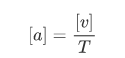Let's put the things together: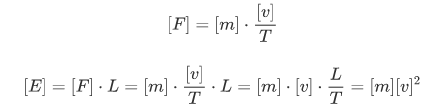So what we have showed is that if you defined energy to be a force times a distance, and the force to be acceleration times mass, then energy will have dimensions of mass times velocity squared. This doesn't mean that we have proved the formula for the kinetic energy or for Einstein's equation E=mc2. But it does mean that whenever we encounter a quantity formed from a mass times a velocity squared, that has dimensions of energy. And we shouldn't be too surprised if we can actually express that quantity as some form of energy.

Dimensional analysis can't prove an equation, but it can help you disprove certain types of equations. By now you should be able to see why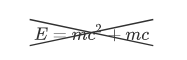or: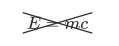can never be acceptable equations.